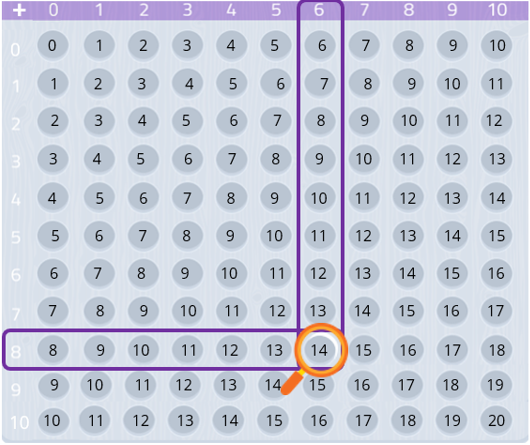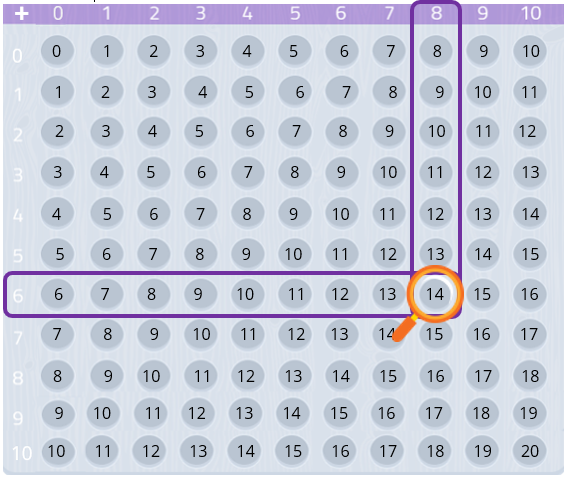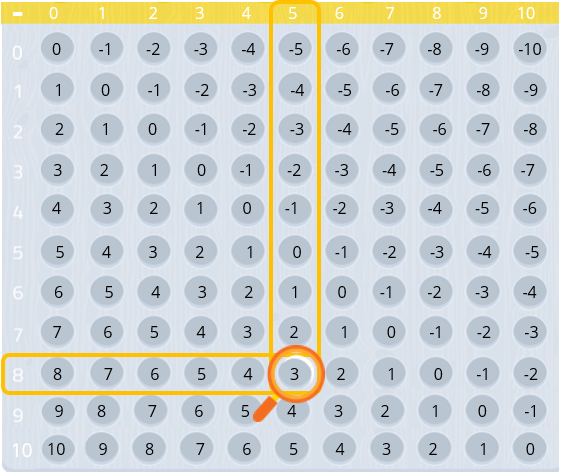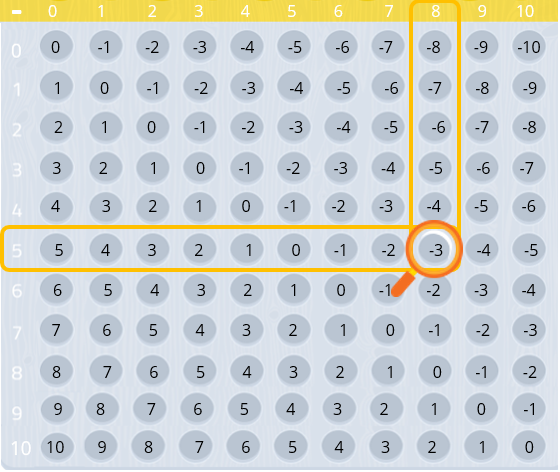Smartick is an advanced online program that teaches kids math and coding in only 15 min. a day

Nov12

# Discovering Subtraction and Addition Tables

For many children, the transition between counting and beginning to add or subtract can be a challenge. We start with our fingers, drawing lines, adding and taking away objects, but perhaps we don’t know that subtraction and addition tables also exist.

These tables, that are numbered from 0, allow us to visually represent the way two numbers are added or subtracted when they intersect on the table, as well as allowing us to discover and understand the properties of each operation. Let’s see how each one works!

1. We choose a number from the left column. For example, the number 8.
2. We choose a number from the top row. For example, the number 6.
3. From the number 8, we move until the square intersects with the number 6. This square indicates the number 14, therefore, this is the answer to our addition problem: 8 + 6 = 14.Steps 1 and 2 can also be done in reverse. This way we can see that it will give us the same result:With the visual help from these tables we can see that:

• The commutative property is fulfilled, 6 + 8 is the same as 8 + 6, therefore, the order of addends does not alter the result.
• If we add 0 to any number, we will always get the same number, because 0 is a neutral element.

#### Subtraction Tables

In the case of subtraction, yes, the order of factors is important because the result of the operation changes, specifically to the sign (positive or negative.) We take the subtraction example of 8 – 5. We locate 8 in the left column and 5 in the top row, the number we find in the square when the two numbers intersect is 3, therefore 8 – 5 = 3.However, if we change the order of the numbers we see that: 5 – 8 = -3.Subtraction displays these properties:

• Changing the order of factors, yes it affects the result, so we say that it does not display the commutative property.
• Subtracting two natural numbers does not always result in a natural number (like in the case of 5 – 8 = -3)
• Subtracting 0 from any number gives the same number as a result because it is a neutral element.

Practice subtraction and addition tables with Smartick so that each day you can get better at math!